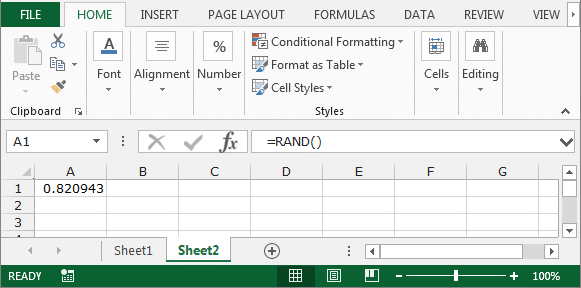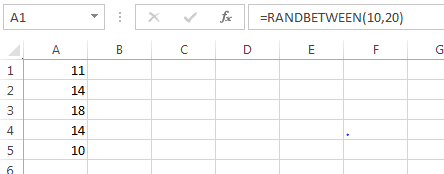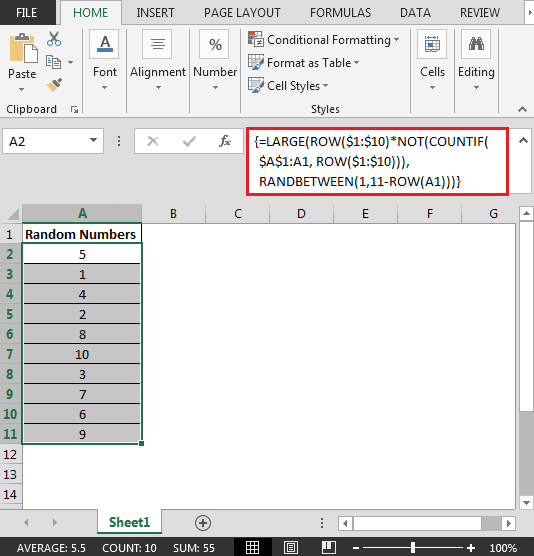# Here we will learn how to generate a random number in Microsoft Excel . To generate a random number, we will use the “RAND” and “RANDBETWEEN” functions.

The rand function is used to generate a random number greater than or equal to 0 and less than 1, and this number will change as each activity on the sheet is performed (the random number changes each time the window is closed and opened).

## How to use the RAND () function: = RAND

for example:

• Select cell A1 and write the function = RAND () inside it.
• Press Enter .
• This function can return a random number.Note: Random numbers change every time we close and reopen the page.

RANDBETWEEN : This function is used to generate a random integer between two specified numbers (between the lower and upper limit). Each time the worksheet is executed, a new random integer is generated.

## How to use the RANDBETWEEN function: (upper limit, lower limit) RANDBETWEEN

• Select cell A1 and write the function (upper limit, lower limit) RANDBETWEEN inside it.
• Press Enter .
• Drag it with the mouse to cell A5 .
• This function generates a random number between 10 and 20.Well, so far you have learned how to create a random number with the Rand and Randbetween functions .

If you want to create a random number without VBA (writing programs and functions in Excel) and by specifying the range (upper and lower limit), you must generate random numbers with the Excel Randbetween function . Let’s talk about how to use this function.

Question: I want to create a list of random numbers without repetition between 1 and 10 in a column. what should I do?

• In cell A2 , write the following formula:

{= LARGE (ROW (\$ 1: \$ 10) * NOT (COUNTIF (\$ A \$ 1: A1, ROW (\$ 1: \$ 10))), RANDBETWEEN (1,11-ROW (A1)))}

• Copy the formula in the range A3: A11 .

Note: This formula is for generating arrays. Keys CTRL + SHIFT + ENTER together to use.• The above formula creates a list of unique random numbers from 1 to 10.

In this method we can use RAND and RANDBETWEEN functions in Excel.